﻿

### On ideal and subalgebra coefficients in a class k-algebras

#### Abstract

Let k be a commutative field with prime field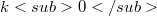$k0$ and A a k- algebra. Moreover, assume that there is a k-vector space basis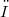$ω$ of A that satisfies the following condition: for all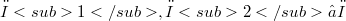$ω1, ω2 ∈ ω$ ,the product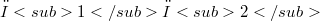$ω1ω2$ is contained in the$k0$-vector space spanned by$ω$. It is proven that the concept of minimal field of definition from polynomial rings and semigroup algebras can be generalized to the above class of (not necessarily associative) k-algebras. Namely, let U be a one-sided ideal in A or a k-subalgebra of A. Then there exists a smallest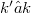$k' ≤ k$ with U-as one-sided ideal resp. as k-algebra—being generated by elements in the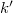$k'$-vector space spanned by$ω$.

DOI Code: 10.1285/i15900932v27n1p77

Keywords: Field of definition; Non-associative k-algebra; One-sided ideal; k-subalgebra

Full Text: PDF PS